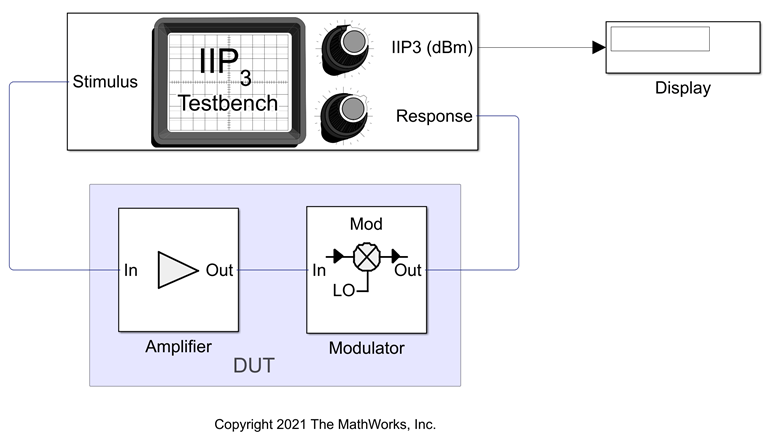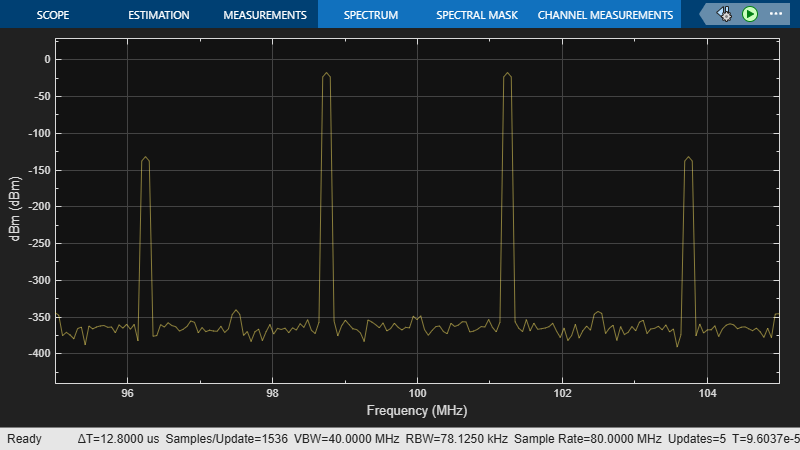# Measure IIP3 of Device Under Test

Use the IIP3 Testbench block to measure the input third-order intercept (IIP3) of device under test (DUT).

Connect the blocks as shown in the model.Set the parameters for DUT and the testbench.

Amplifier block:

• Available power gain`10` dB

• Intercept points convention`Input`

• IP3`32` dBm

Mixer block:

• Available power gain`5` dB

• Local oscillator frequency`2.0` GHz

• Add Image Reject filter`on`

• Intercept points convention`Input`

• IP3`35` dBm

• Filter type`Highpass`

• Implementation`Constant per carrier`

• Passband edge frequency`2.0` GHz

IIP3 Testbench block:

• Input frequency (Hz)`2.1e9`

• Output frequency (Hz)`0.1e9`

• Simulate noise (both stimulus and DUT internal)`off`

Run the model. You will see that the display shows an IIP3 value of `24.19` dBm. This value can be verified analytically using the equation provided in .

IIP3 of the DUT = – 10*log10(1/10^((32)/10) + 10^(10/10)/10^((35)/10)) = 24.2099 dBm

where,

IIP3 of the amplifier in linear scale = 10^((32)/10)

IIP3 of the mixer in linear scale = 10^((35)/10)

Available power gain of the amplifier = 10^(10/10)Razavi, Behzad. “Basic Concepts “ in RF Microelectronics, 2nd edition, Prentice Hall, 2012.